Type
Solution Manual
Book Title
Fundamentals of Investments: Valuation and Management 8th Edition
ISBN 13
978-1259720697

### 978-1259720697 Chapter 20 Solution Manual

January 2, 2020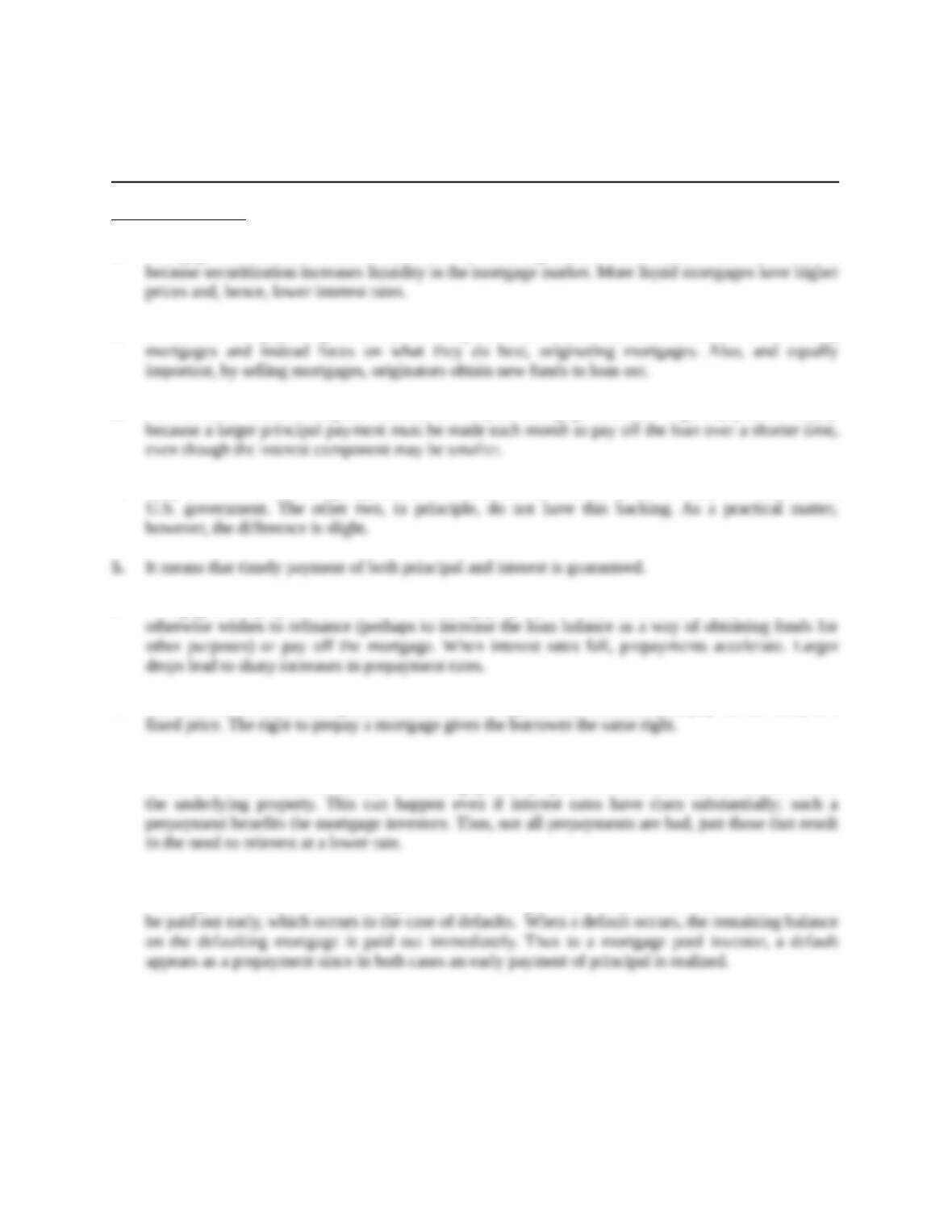Chapter 20
Mortgage-Backed Securities
Concept Questions
1. Mortgage securitization benefits borrowers by reducing interest rates. Interest rates are reduced
2. It benefits mortgage originators by allowing them to transfer the risk associated with holding
3. For the same rate and original balance, the 15-year mortgage will have higher payments simply
4. Only GNMA is a federal agency, and GNMA securities are backed by the full faith and credit of the
6. Mortgages are prepaid because the underlying property is sold, interest rates fall, or the owner
7. The call feature on a bond gives the borrower the right to buy the bond (i.e., pay off the debt) at a
8. Prepayments that result purely from interest drops are a risk; the mortgage investor will have to
reinvest at a lower rate. However, some mortgages are prepaid for other reasons, such as the sale of
9. For a fully modified mortgage pool, all cash flows are guaranteed to be paid in a timely manner,
meaning that no cash flows will be paid out late. The guarantee does, however, allow cash flows to
Education.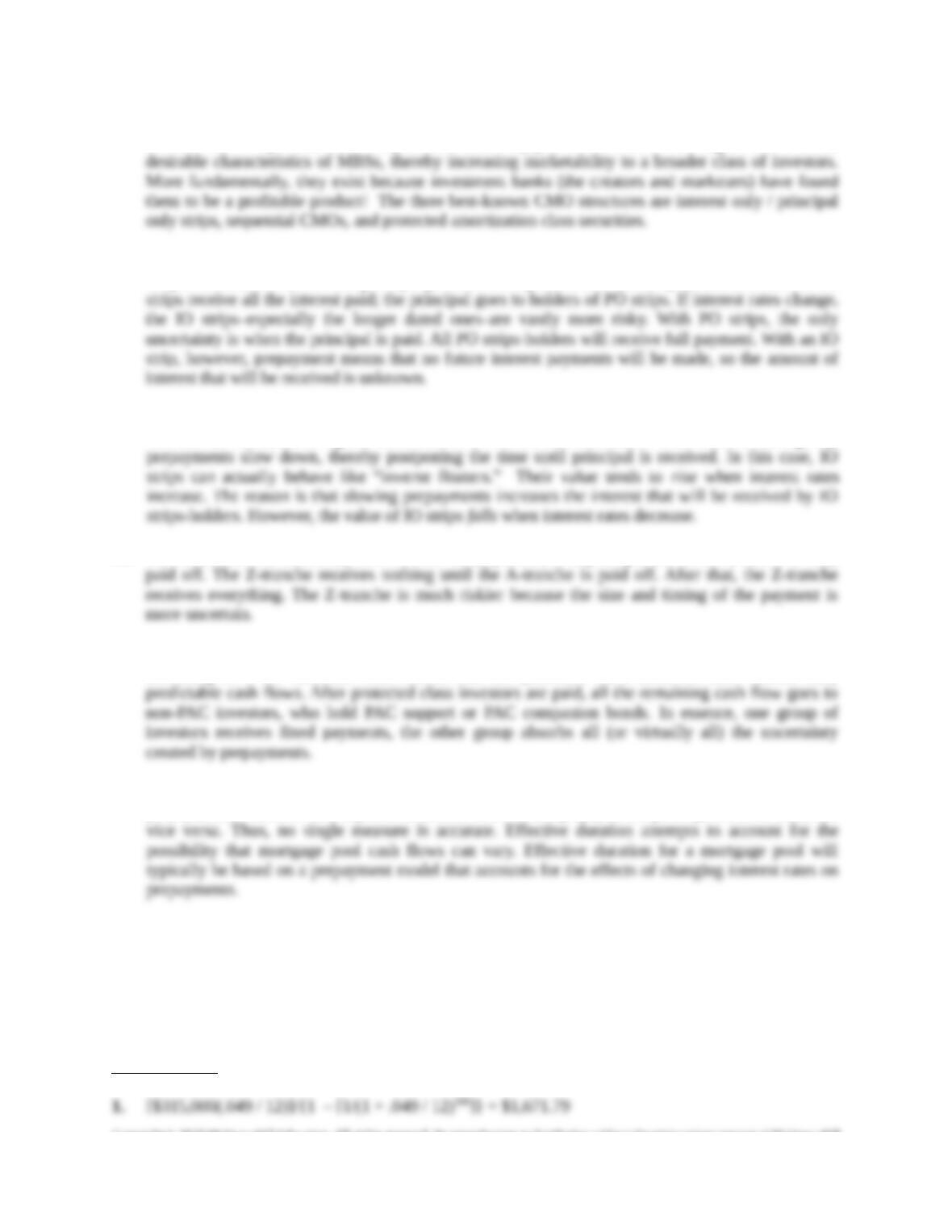10. A collateralized mortgage obligation (CMO) is a mortgage-backed security with cash flows that are
divided into multiple securities. They exist because they provide a means of altering some of the less
11. Every mortgage payment has an interest portion and a principal portion. IO and PO strips are very
simple CMOs; the interest and principal portions are separated into distinct payments. Holders of IO
12. PO strips have greater interest rate risk if we define interest rate risk to mean losses associated with
interest rate increases and gains associated with interest rate decreases. When interest rates go up,
13. The A-tranche will essentially receive all of the payments, both principal and interest, until it is fully
14. With a protected amortization class (PAC) CMO, payments are made to one group of investors
according to a set schedule. This means that the protected class investors have almost fully
15. Macaulay duration assumes fixed cash flows. With MBSs and CMOs, the payments depend on
prepayments, which in turn depend on interest rates. When prepayments pick up, duration falls, and
Solutions to Questions and Problems
NOTE: All end of chapter problems were solved using a spreadsheet. Many problems require multiple
steps. Due to space and readability constraints, when these intermediate steps are included in this
solutions manual, rounding may appear to have occurred. However, the final answer for each problem is
found without rounding during any step in the problem.
Core Questions
Education.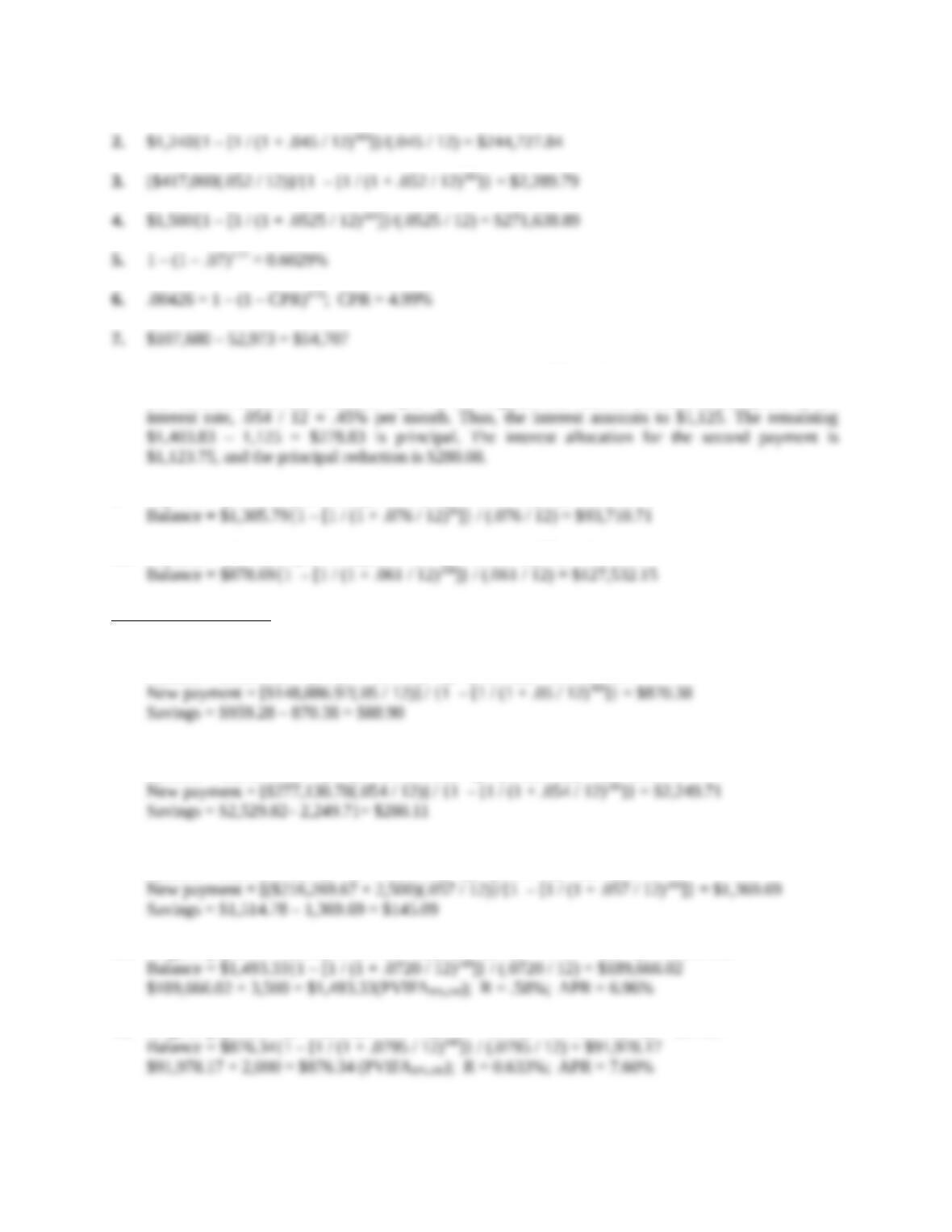8. Payment = [\$250,000(.054 / 12)] / {1 – [1 / (1 + .054 / 12)360]} = \$1,403.83
The interest in the first month is equal to the original loan amount (\$250,000) multiplied by the
9. Payment = [\$140,000(.076 / 12)] / {1 – [1/(1 + .076 / 12)180]} = \$1,305.79
10. Payment = [\$145,000(.061 / 12)]/{1 – [1 / (1 + .061 / 12)360]} = \$878.69
Intermediate Questions
11. Original payment = [\$160,000(.06 / 12)]/{1 – [1 / (1 + .06 / 12)360]} = \$959.28
Balance = \$959.28{1 – [1 / (1 + .06 /12)300]} / (.06 / 12) = \$148,886.97
12. Original payment = [\$350,000(.0725 / 12)] / {1 – [1 / (1 + .0725 / 12)300]} = \$2,529.82
Balance = \$2,529.82{1 – [1 / (1 + .0725 / 12)180]} / (.0725 / 12) = \$277,130.78
13. Original payment = [\$230,000(.0690 / 12)] / {1 – [1 / (1 + .0690 / 12)360]} = \$1,514.78
Balance = \$1,514.78{1 – [1 / (1 + .069 / 12)300]} / (.069 / 12) = \$216,269.67
14. Original payment = [\$220,000(.0720 / 12)]/{1 – [1 / (1 + .0720 / 12)360]} = \$1,493.33
15. Original payment = [\$120,000(.0795 / 12)] / {1 – [1 / (1 + .0795 / 12)360]} = \$876.34
Education.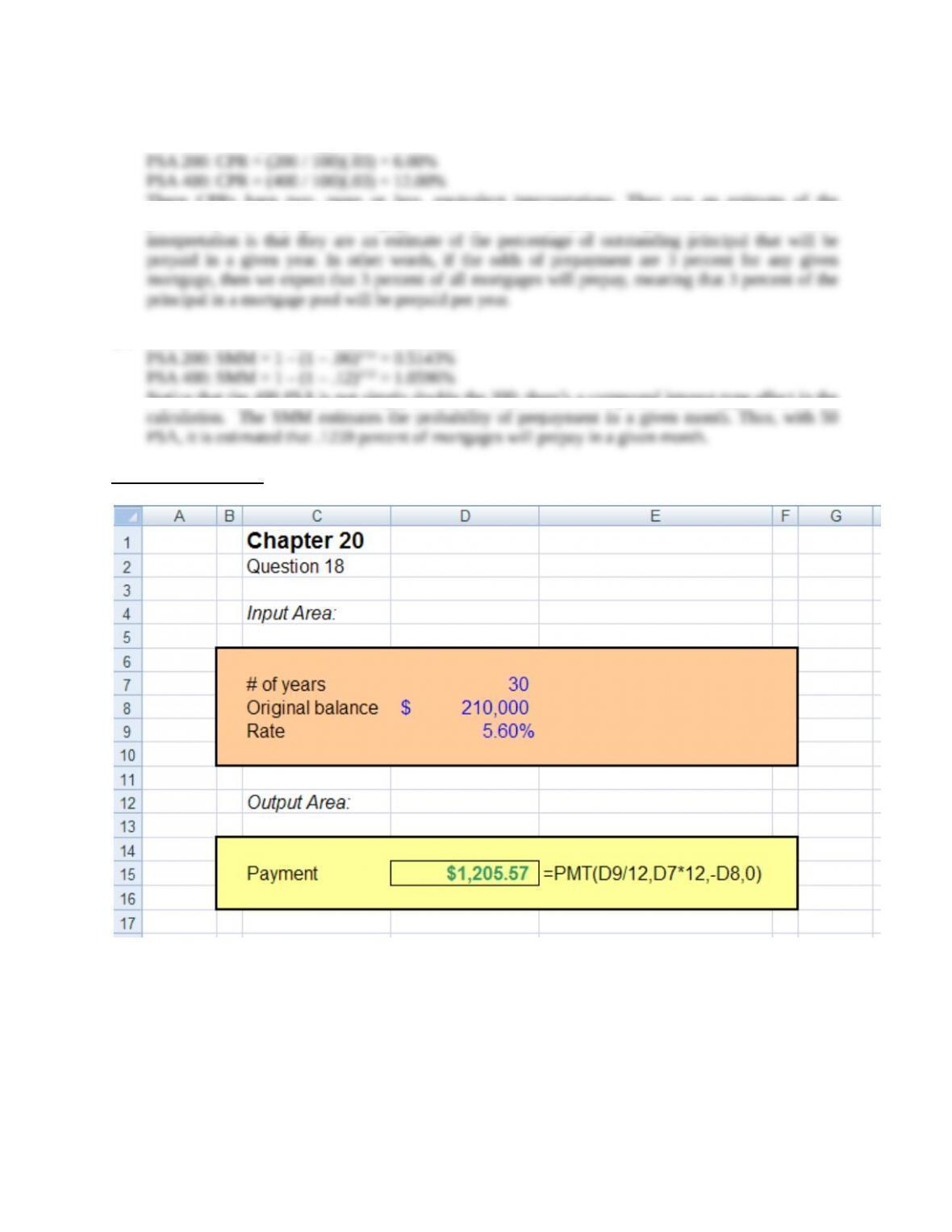16. For a seasoned 100 PSA mortgage, the CPR is 3 percent per year.
PSA 50: CPR = (50 / 100)(.03) = 1.50%
These CPRs have two, more or less, equivalent interpretations. They are an estimate of the
probability that any given mortgage in the pool will prepay in a given year. A more useful
17. PSA 50: SMM = 1 – (1 – .015)1/12 = 0.1259%
Notice that the 400 PSA is not simply double the 200; there’s a compound interest-type effect in the
Education.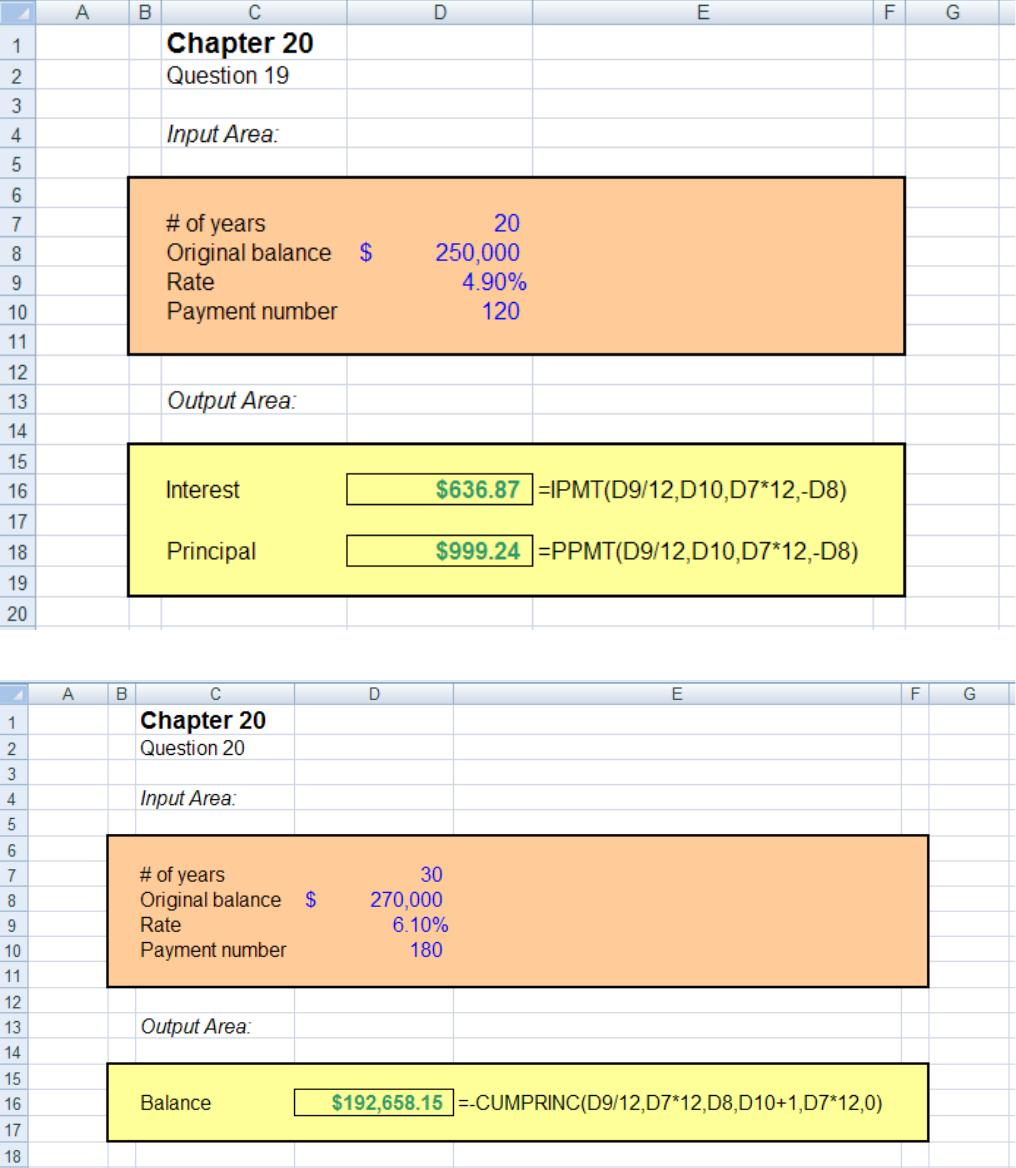Education.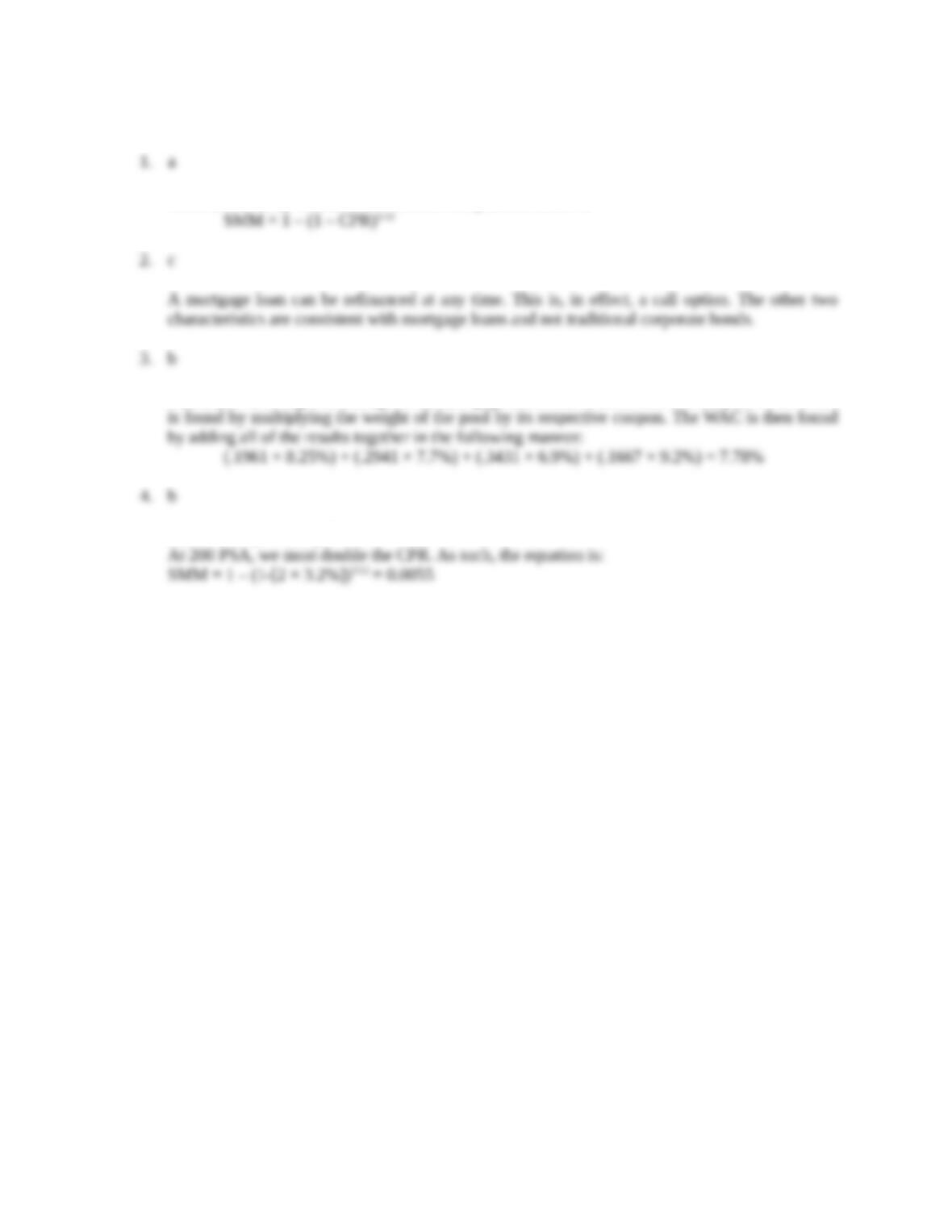CFA Exam Review by Kaplan Schweser
CPRs are industry benchmarks. SMM is computed as follows:
This is just the weighted average of the mortgage rates. The contribution of each pool to the WAC
SMM = 1 – (1 – CPR)1/12